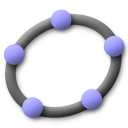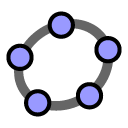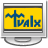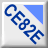6.0.590

File size:
64.92 MB
File name:
GeoGebra-Windows-Installer-6-0-590-0.exe
Virus/malware test:
Clean
Operating system:
Windows x32/x64

## Alternative softwareSmart Math Calculator
Define variables and solve mathematical equations and functions.Math Calculator
It enables you to calculate expressions and solve equations.GeoGebra Classic
A collection of mathematical tools for algebra, calculus and geometry.Math Center Level 1
The program helps you study graphics, make calculations and solve equations.Abacus Math Writer
For publishing Mathematical and Scientific equations.Calculator Vaxa CE-82e
Scientific calculator to solve equations.